### Active control of the flow past a cylinder using deep reinforcement learning

Andre Weiner, Fabian Gabriel, Darshan Thummar
TU Braunschweig, Institute of Fluid MechanicsDarshan Thummar: Active flow control in simulations of fluid flows based on DRL, Github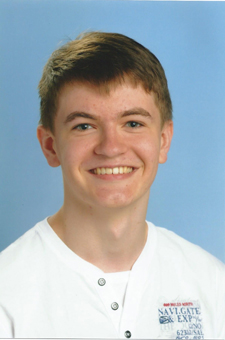Fabian Gabriel: Active control of the flow past a cylinder under Reynolds number variation using DRL, Github

## Outline

1. Flow control problem
2. Proximal Policy Optimization (PPO)
3. Implementation in OpenFOAM and PyTorch
4. Selected results
5. Summary and outlook

6. OpenFOAM committee on data-driven modeling

## Controlling the flow past a cylinder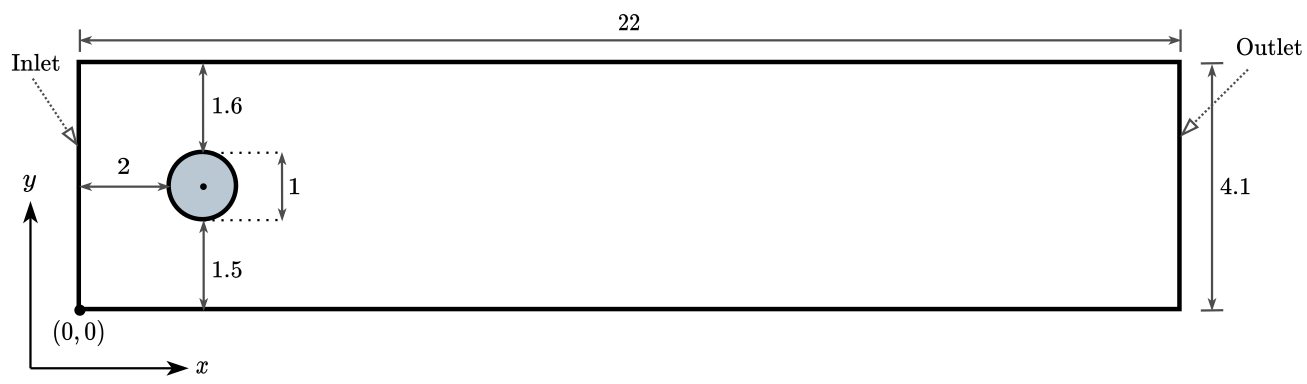Based on M. Schäfer, S. Turek (1996); $Re=100$; 2D; pimpleFoam.

Flow past a circular cylinder at $Re=100$ - without control.

Can we reduce drag and lift forces?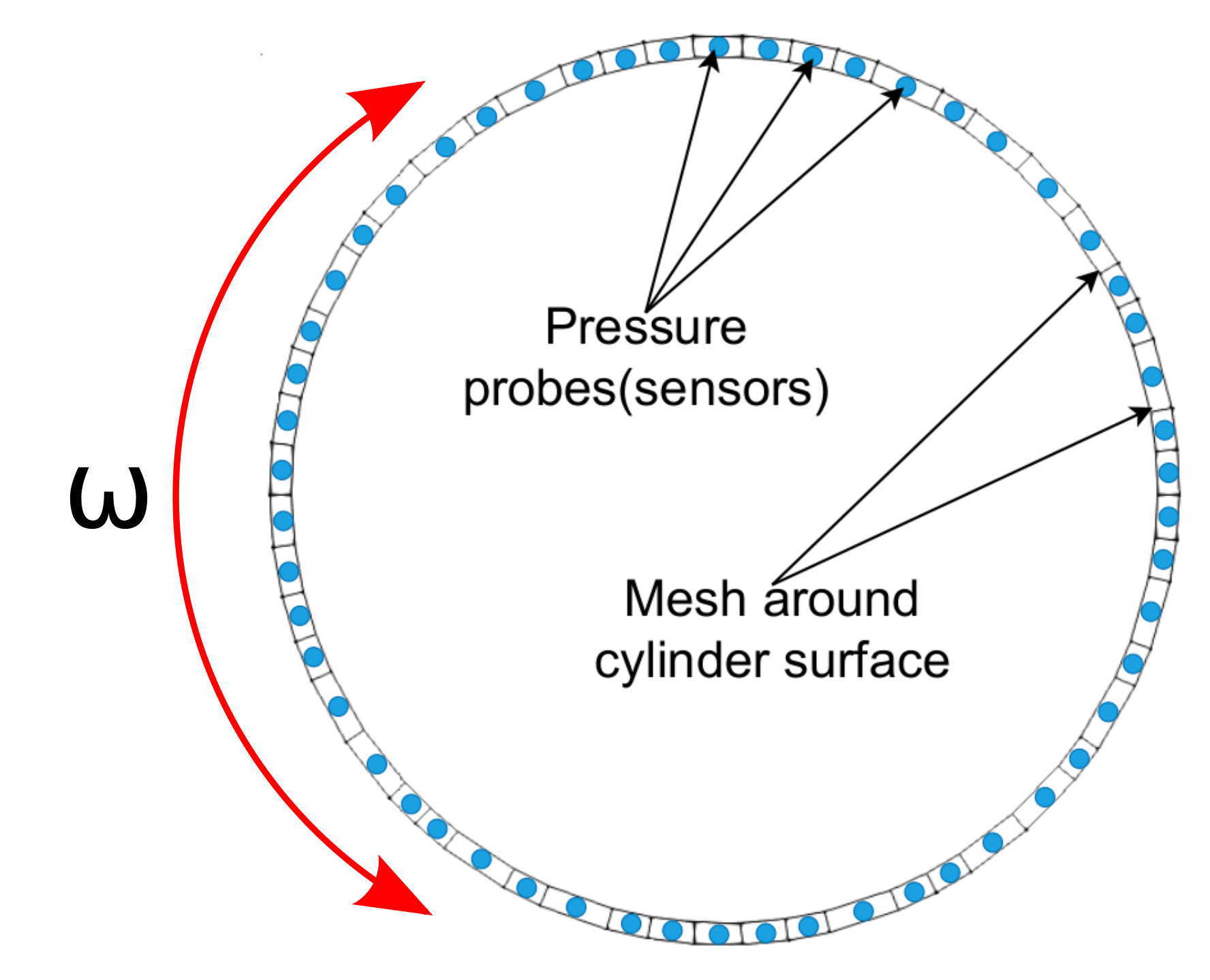## Proximal Policy Optimization (PPO)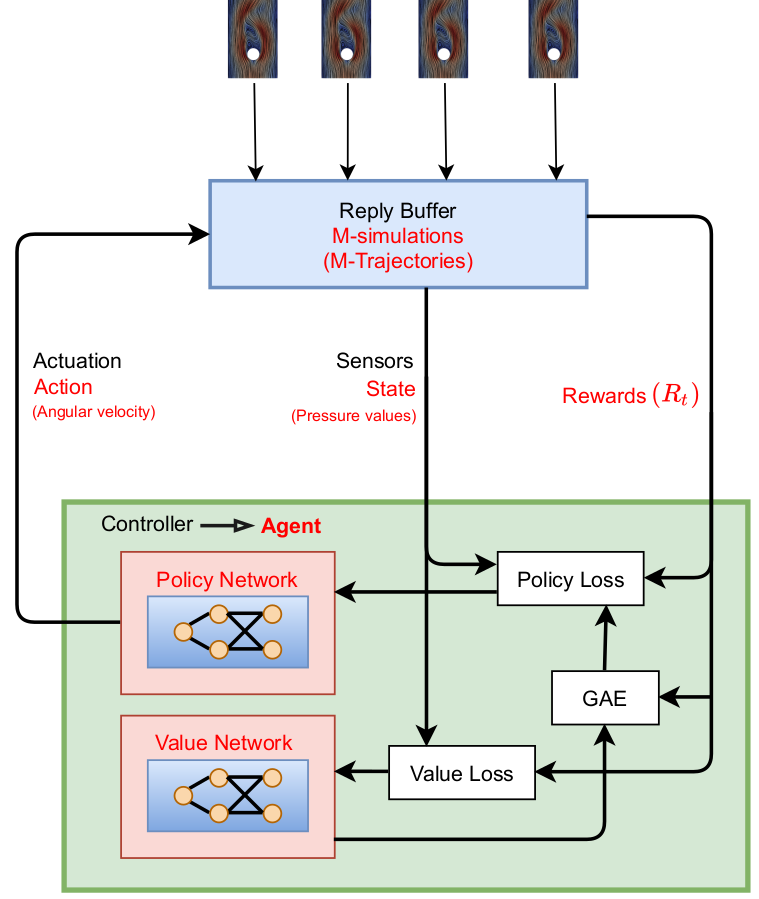Proximal policy optimization (PPO) workflow (GAE - generalized advantage estimate).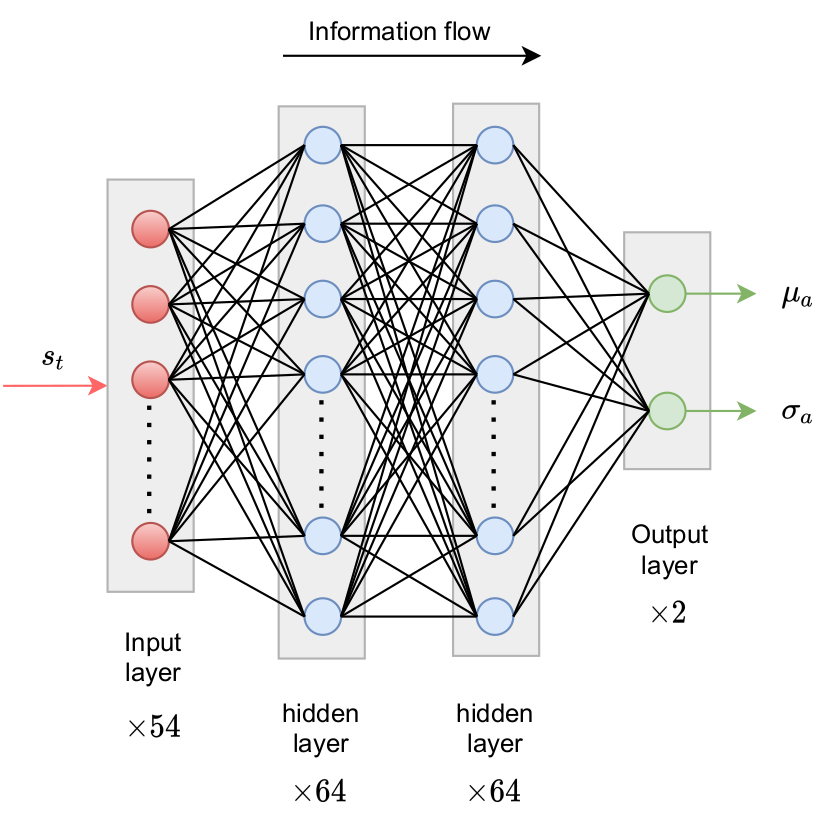Policy networks outputs parameters of probability density function.

reward at time $t$

$$R_t = r_0 - \left( r_1 c_D + r_2 |c_L| + r_3 |\dot{\theta}| + r_4 |\ddot{\theta}| \right)$$

• $c_D$ - drag coefficient
• $c_L$ - lift coefficient
• $\dot{\theta}$ - angular velocity
• $\ddot{\theta}$ - angular acceleration
• $r_i$ - constants

long-term consequences

$$G_t = \sum\limits_{l=0}^{N_t-t} \gamma^l R_{t+l}$$

• $t$ - control time step
• $G_t$ - discounted return
• $\gamma$ - discount factor, typically $\gamma=0.99$
• $N_t$ - number of control steps

learning what to expect in a given state - value function loss

$$L_V = \frac{1}{N_\tau N_t} \sum\limits_{\tau = 1}^{N_\tau}\sum\limits_{t = 1}^{N_t} \left( V(s_t^\tau) - G_t^\tau \right)^2$$

• $\tau$ - trajectory (single simulation)
• $s_t$ - state/observation (pressure)
• $V$ - parametrized value function
• clipping not included

Was the selected action a good one?

$$\delta_t = R_t + \gamma V(s_{t+1}) - V(s_t)$$ $$A_t^{GAE} = \sum\limits_{l=0}^{N_t-t} (\gamma \lambda)^l \delta_{t+l}$$

• $\delta_t$ - one-step advantage estimate
• $A_t^{GAE}$ - generalized advantage estimate
• $\lambda$ - smoothing parameter

make good actions more likely - policy objective function

$$J_\pi = \frac{1}{N_\tau N_t} \sum\limits_{\tau = 1}^{N_\tau}\sum\limits_{t = 1}^{N_t} \left( \frac{\pi(a_t|s_t)}{\pi^{old}(a_t|s_t)} A^{GAE,\tau}_t\right)$$

• $\pi$ - current policy
• $\pi^{old}$ - old policy (previous episode)
• clipping and entropy not included
• $J_\pi$ is maximized

### Why PPO?

• continuous and discrete actions spaces
• relatively simple implementation
• sample efficient
• ...

Refer to R. Paris et al. 2021 and the references therein for similar works employing PPO.

### How long does it take?

$$t_{train} \approx t_{sim,single} \times N_{episodes}$$

current cylinder example:

$$t_{train} \approx 0.5h \times 50 = 25h$$

### How expensive is it?

$$t_{cpu} = t_{train} \times N_{cpu, single} \times N_\tau$$

current cylinder example:

$$t_{cpu} = 25h \times 4 \times 10 = 1000h$$

## Implementation in OpenFOAM and PyTorch

Python/PyTorch

1. create policy and value networks
2. fill trajectory buffer (run simulations)
3. update policy and value networks
4. go back to 1. until converged

Implementation follows closely chapter 12 of Miguel Morales's Grokking Deep Reinforcement Learning

C++/OpenFOAM/PyTorch

2. sample and apply action
3. write trajectory (state-action pairs)

Boundary condition defined in 0/U


cylinder
{
type            agentRotatingWallVelocity;
// center of cylinder
origin          (0.2 0.2 0.0);
// axis of rotation; normal to 2D domain
axis            (0 0 1);
// name of the policy network; must be a torchscript file
policy          "policy.pt";
// when to start controlling
startTime       0.01;
// how often to evaluate policy
interval        20;
// if true, the angular velocity is sampled from a Gaussian distribution
// if false, the mean value predicted by the policy is used
train           true;
// maximum allowed angular velocity
absOmegaMax     0.05;
}


## Selected results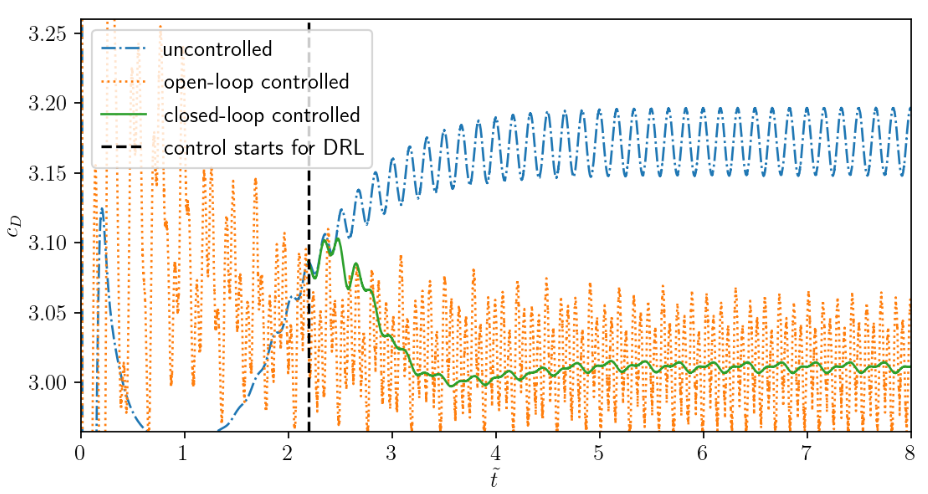Comparison of uncontrolled, open-loop controlled, and closed-loop controlled drag.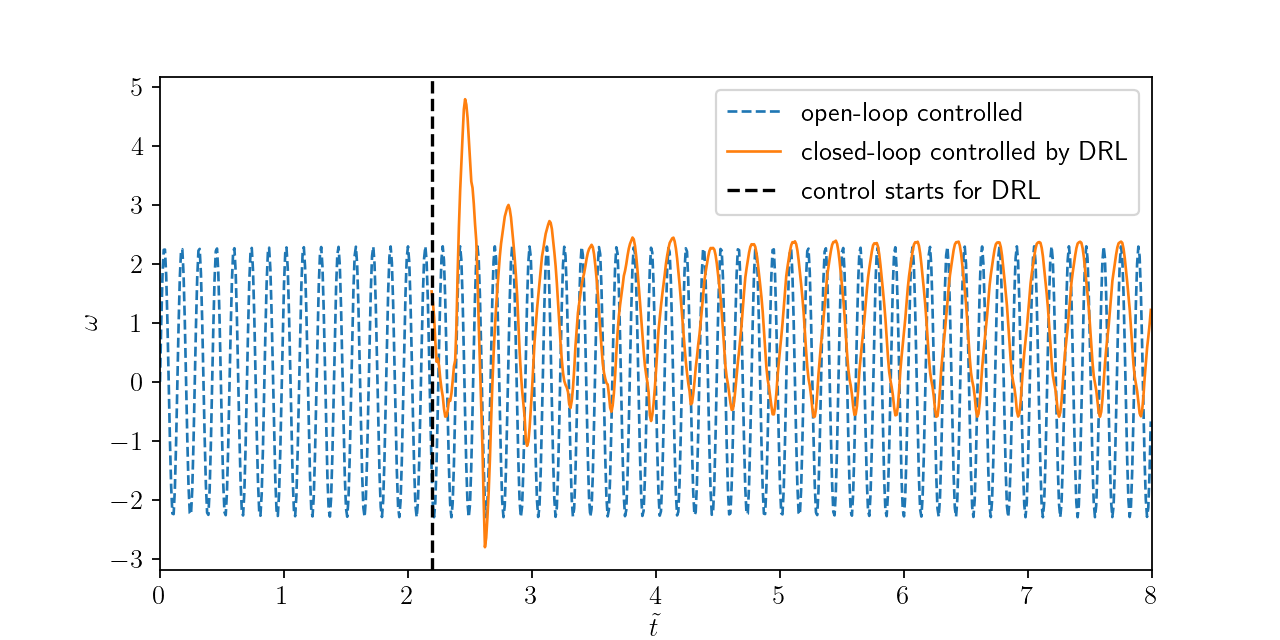Angular velocity for open and closed-loop control.

### How robust is the controller?

1. training with steady inlet velocity
$Re=\{100, 200, 400 \}$
2. test with unsteady inlet velocity
$Re(t)= 250 + 150\mathrm{sin}(\pi t)$

Variable inlet velocity/Reynolds number $Re(t) = 250 + 150\mathrm{sin}(\pi t)$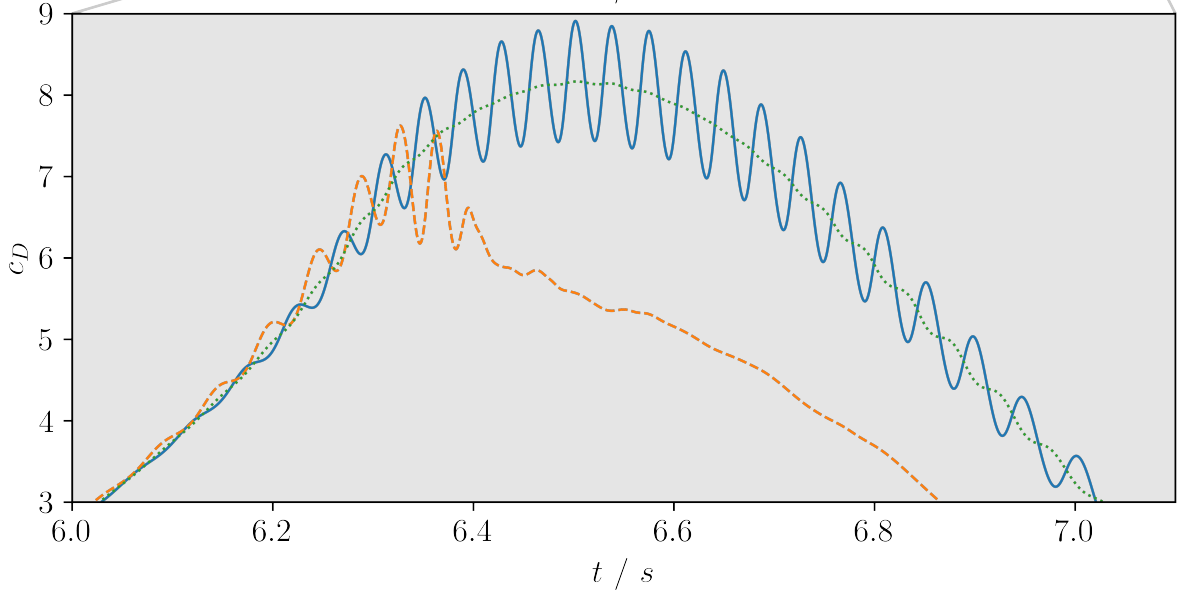Drag coefficient for transient inlet velocity: uncontrolled and controlled.

## Summary

• DRL solves end-to-end control problem
• learned control law appears to be robust
• DRL for real applications is in reach with
OpenFOAM+PyTorch+HPC

## Outlook

• extend/modify state (observation)
• model-based PPO extension
• benchmark closed-loop control algorithms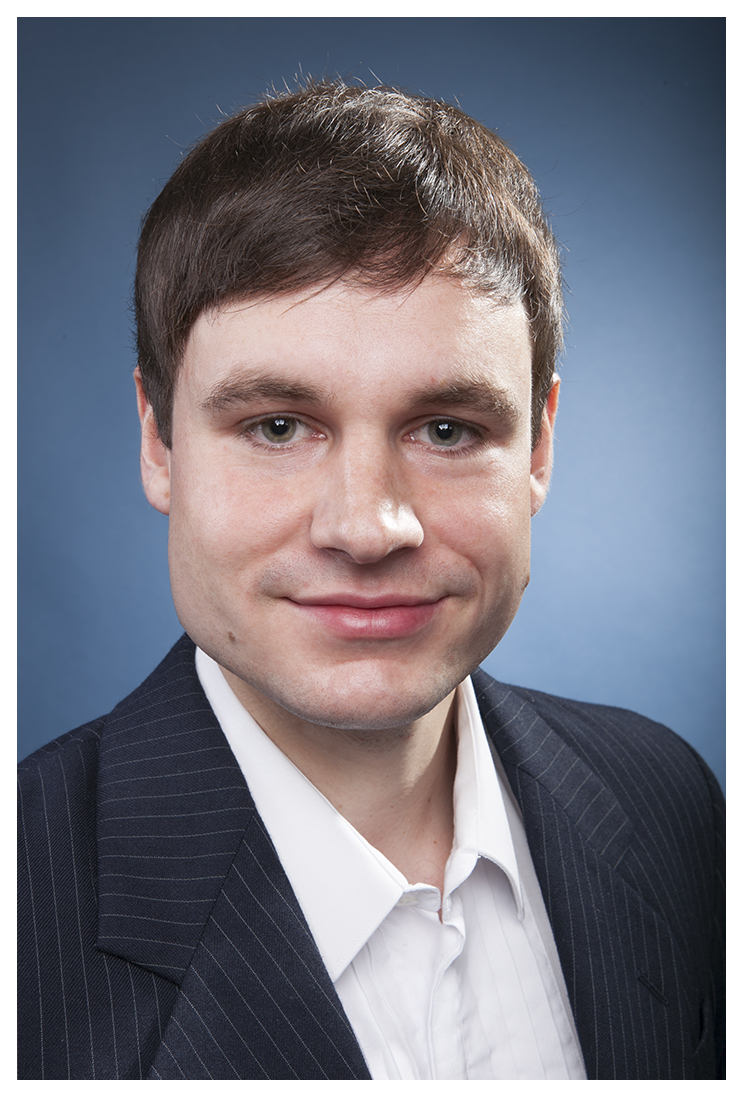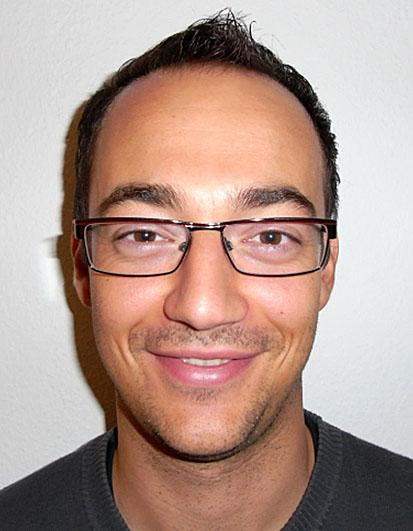Andre Weiner Tomislav Marić a.weiner@tu-braunschweig.de maric@mma.tu-darmstadt.de